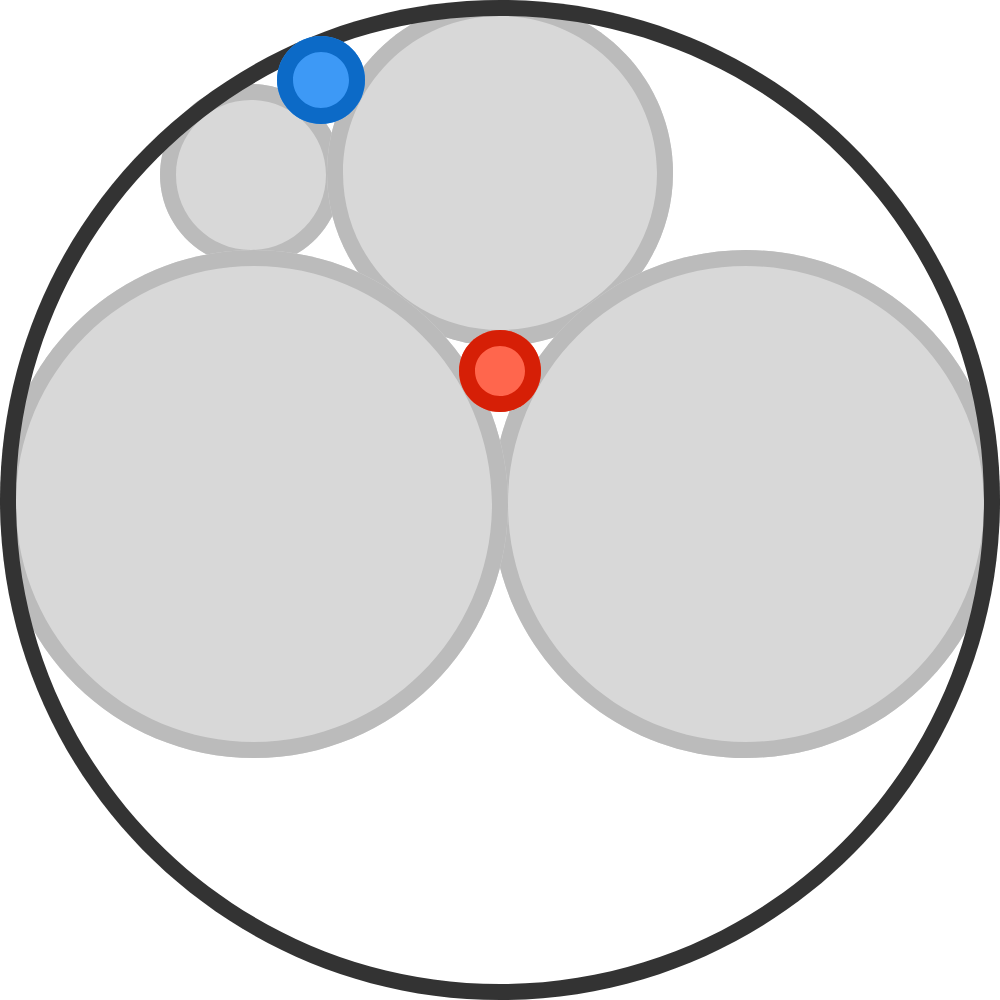# Mind The Gaps

Geometry Level 3The two large gray circles are congruent, and each is half the diameter of the largest circle. All circles that appear to be tangent to each other are indeed tangent to each other.

$\frac{\text{radius of blue circle}}{\text{radius of red circle}} = \frac{a}{b},$
where $a$ and $b$ are coprime positive integers. Find $a+b$.

×